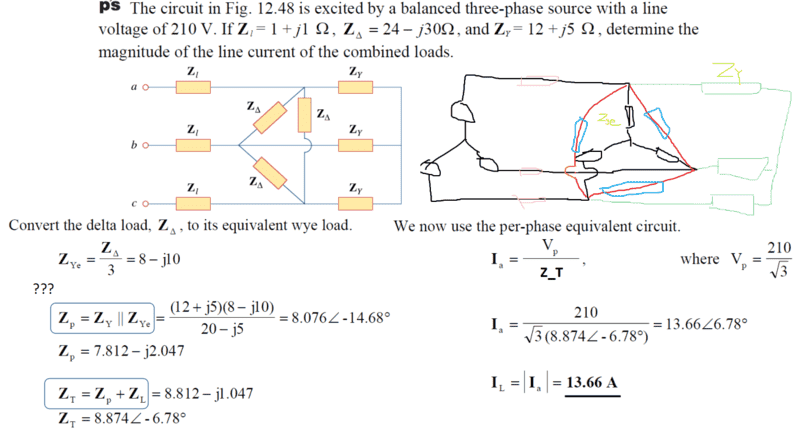# Three-phase circuit: Why Z_Ye is parallel with Z_Δ?

• Engineering

## Homework Statement

This problem is taken from Fundamentals of Electric Circuits (chapter 12, problem 15).## Homework Equations

As shown in the first blue box, I have trouble seeing why after the conversion, Z_Ye will be parallel with Z_Y. I think it's because the voltages 'emitting' from the converted network is like another 3-phase voltage source.

## The Attempt at a Solution

The diagram on the right-hand side is my sketch in Microsoft Paint, where I fail to see why are them parallel after the conversion.

#### Attachments

Last edited:

NascentOxygen
Staff Emeritus
Perhaps you should introduce a 4th line, the neutral. See whether you can draw the Y network (in place of the ∆ arrangement) when you have a neutral line available.

(I suggest that you self-impose a constraint of no oblique lines; draw all lines in your Y network horizontally or vertically.)

I think I disagree with what NascentOxygen just posted. The circuit doesn't require a 4th wire to handle the neutral point.

If you draw a wye inside a delta, the neutral point of the wye will be sufficient without the addition of a neutral wire.

The Electrician
Gold Member
It's true that the circuit doesn't require a 4th wire, but the OP is having trouble seeing why the two wye subcircuits are in parallel. Adding a wire between the center nodes of the pre-existing wye (composed of Zy) and the wye resulting from the delta-wye conversion of ZΔ will make it clear why each element of one wye is in parallel with the corresponding element of the other wye. This wire will carry no current because of the symmetry, so adding it doesn't change the behavior of this very symmetrical circuit. In a circuit without this symmetry, adding this 4th wire would probably change the circuit behavior.

•NascentOxygen